# TLBO Optimization Algorithm Based-Type2 Fuzzy Adaptive Filter for ECG Signals Denoising

TLBO Optimization Algorithm Based-Type2 Fuzzy Adaptive Filter for ECG Signals Denoising
LAAS Laboratory, Electronics Department, Faculty of Technology, University of M’sila, M’sila 28000, Algeria

GREYC Laboratory, Caen University, Caen 14000, France

LAAAS Laboratory, Electronics Department, Faculty of Technology, University of Batna 2, Batna 5000, Algeria

Corresponding Author Email:
mohammedassam.ouali@univ-msila.dz
Page:
541-553
|
DOI:
https://doi.org/10.18280/ts.370401
3 May 2020
|
Accepted:
26 July 2020
|
Published:
10 October 2020
| Citation

OPEN ACCESS

Abstract:

A novel type2-fuzzy adaptive filter is presented, which uses the concepts of type2-fuzzy logic, for electrocardiogram signals denoising. Type2-fuzzy adaptive filter is an information processor where both numerical and linguistic information are used as input-output pairs and fuzzy if-then rules, respectively. The proposed approach is based on an iterative procedure to achieve acceptable information extraction in the case where the statistical characteristics of the input-output signals are unknown. The proposed filter is presented as a dual-layered feedback system. Each layer has different function, the first layer being the type2-fuzzy autoregressive filter model. The second layer being responsible for training the membership function parameters. The second layer adjusts the type2-fuzzy adaptive filter parameters by using a teaching learning-based optimization algorithm (TLBO), which will allow the reaching of the required signal reconstruction by decreasing the criterion function. The proposed filter is validated and evaluated through some experimentations using the MIT-BIH ECGs databases where various artifacts were added to the ECGs signals; these included real and artificial noise. For comparison purposes, both model and non-model-based methods recently published are used. Furthermore, the effect of the proposed filter on the malformation of diagnostic features of the ECG was studied and compared with several benchmark schemes. The results show that the proposed method performs better denoising for all noise power levels and for a different criteria viewpoint.

Keywords:

ECG signal, ECG denoising, type-2 fuzzy logic, optimization algorithm, TLBO

1. Introduction

The purpose of an electrocardiogram (ECG) is to measure electrical potential changes in the heart over a specific time period. Particular cells in the heart produce electrical impulses that spread through the heart causing it to contract, thereby controlling the rate of the heart’s beating and causing these changes in the electrical potentials [1-4]. In the ECG, these electrical changes are measured through electrodes positioned on the chest. The electrical impulses are recorded in millivolts. Whilst the idealized heartbeat comprises several complexes, three of these complexes are frequently recorded and utilized in medical ECGs Terminology. These are the P complex, which measures the depolarization of the atrium, the QRS complex, which measures the depolarization of the ventricles, and the T wave, measuring the repolarization of the ventricles. However, the ECG signal frequently becomes contaminated by both internal and external noises. Whilst there are numerous sources of these noises, the ones of primary interest are instrument and Electromyogram (EMG) noises, electrode contact noise, motion artifacts and power line interference . As such, it is necessary to carry out advanced digital processing of the ECG waveforms in order to use the ECG of an individual for identification and diagnosis purposes. Obviously, any noise that appears on the trace of the ECG can complicate diagnosis and identification analysis. Therefore, it is necessary to understand and cancel out the effects of noise from an ECG trace in order to extract the required identifying features of the trace itself. The required identification data can be masked by artifacts introduced by noise. Since its conception, outnumber of methods have been used to denoise ECG signals. The most widely used methods for ECG denoising are the non-model-based methods including a hybrid denoising scheme to enhance ECG signals by combining high-order synchro squeezing transform (FSSTH) with non-local means (NLM) , dual-threshold filter and discrete wavelet transform (ADTF-DWT) , the empirical mode decomposition and genetic algorithm for adaptive denoising  and the adaptive Fourier decomposition (AFD) . Other contributions in this subject are reported in Wang et al. study [10-13] which have been widely used for ECG denoising. Alternatively, a model-based method has been proposed, McSharry et al. use a three-state non-linear dynamical model in Cartesian space for the generation of synthetic ECG signals. Indeed, several papers have used this model in order to denoise the ECG signal . Hesar and Mohebbi  used this model with Bayesian filtering framework called the marginalized particle-extended Kalman filter (MP-EKF) for ECG denoising purposes. This model was extensively used by several researchers as reported by Sameni et al. [16, 17].

It has been proved that Fuzzy systems are universal approximators, i.e., that an arbitrary continuous function can be approximated within any given precision. To be more precise, it has been proven that a system represented by fuzzy rules can approximate any real system given by its input-output data, with any given accuracy. The construction of the fuzzy system encompasses two steps : The first one is concerned with the construction of the fuzzy system model’s structure. Several contributions in this subject are reported in the papers [19-23]. The second step is the identification of the constructed fuzzy system model’s parameters using a set of input-output data pairs. Many methods have been discussed in the literature to identify such parameters including gradient descent, nonlinear least squares, and Kalman filter [18-23]. Chafaa et al.  have proposed an efficient approach to construct automatically a Takagi-Sugeno fuzzy models where the free parameters were adjusted using the Kalman filter algorithm twice. dos Santos Coelho and Herrera  developed a method for identifying a Takagi-Sugeno fuzzy model based on the chaotic particle Swarm optimization algorithm combined with an efficient Gustafson-Kessel clustering algorithm. Fuzzy Wiener model was proposed by Li and Yang  to identify chaotic systems, where the model’s parameters were tuned using a particle swarm optimization algorithm. Abiyev et al.  in their work presented a new Type2-Fuzzy-neuro system to detect equalizing time-varying channels and detect time-varying systems, where the identification step was achieved using clustering and gradient algorithms.

Type-2 fuzzy sets are extension of the ordinary type-1 fuzzy sets, which are defined by membership functions which are themselves fuzzy. The major advantage of type-2 fuzzy systems is that they can produce reasonable outputs when ambiguity and imprecision occur. Fuzzy logic systems have many sources of uncertainty, such as (1) uncertainties of their inputs (uncertainties in the premise membership functions); (2) uncertainties of their outputs (uncertainties in the consequence membership functions) and (3) linguistic uncertainties, where the significance of the terms used in the antecedent and consequence parts may means different things to various people.

Meta-heuristic algorithms are well suited for solving difficult problem of optimization where they are used successfully. They are often applied to several fields in different areas. In such algorithms, the optimal solution is obtained by parallel processing in the population. These techniques are frequently inspired by biological or biogeographical mechanisms. In this investigation, Teaching Learning-Based Optimization (TLBO), is used in order to tune the type-2 fuzzy filter free parameters adaptively. The TLBO algorithm is inspired by the teaching-learning process and based on the effect of the teacher on the students in the classroom.

Efficiency of combining meta-heuristic optimization methods and fuzzy logic have been exhaustively analyzed and reported by Olivas et al. [24-27]. This investigation proposes an effective electrocardiogram signal filtering method based on type2-fuzzy logic and Teaching Learning Based-Optimization algorithm. Four stages are needed to construct the proposed TLBO based type-2 fuzzy adaptive filter. (1) In the first stage we define the initial membership functions of the filter input and output which must cover the entire input space. (2) In the second stage we construct a set of tunable type2-fuzzy rules based on numerical information extracted from training input-output data pairs. (3) In the third stage, the rules of the filter are constructed. (4) and last, in the fourth step we update the free parameters of the filter using the TLBO algorithm.

The free parameters of type-2 fuzzy filter to be tuned by the TLBO algorithm are the Gaussian centres and widths of the type-2 fuzzy premise membership functions and the consequence intervals. This approach is evaluated through intensive computer experimentations using MIT-BIH ECGs database. It is envisaged that this method will successfully deal with white Gaussian (WG) noises and the real noises (MA and EM) that are taken from the MIT-BIH noise stress test database . By comparing the results of this method with those of other benchmark methods (FSSTH-NLM, ADTF-DWT, AFD, EEMD, and MP-EKF), it can be seen statistically that the proposed technique has a significant performance improvement.

The rest of this paper is structured as follows: after section1; section 2 presents an overview of the type-2 fuzzy system and TLBO algorithm. Section 3 outlines the proposed technique. Simulations and discussions are presented in section 4. Section 5 concludes the paper.

2. Preliminaries

As mentioned previously, the concepts of fuzzy logic [29-33] was extended to type-2 fuzzy logic. In our work, Type-2 fuzzy logic will be used to design adaptive filters. The proposed filter combines the set of input-output measurement pairs with a set of fuzzy If-Then rules. The benefits of using a type2-fuzzy adaptive filter are its non-linear nature and simple design. This simplicity allows human experts to incorporate linguistic information into the filter. When there is no linguistic information available, the type-2 fuzzy adaptive filters can be re-defined as non-linear adaptive filters. The parameters of the membership functions are tuned with an adaptive procedure to characterize the If-Then rules through the use of some criterion function.

A dual-layered feed-forward network structure can be used to represent the type-2 fuzzy adaptive filter [34-36]. Therefore, it will be possible to train the type-2 fuzzy adaptive filter to determine the required input-output relationship through the use of some learning algorithms, such as the TLBO in this investigation. Within this study, the type-2 fuzzy adaptive filter's input $([x(k), x(k-1), \ldots, x(k-n+1)])$ is equal to the previous output, therefore the new input $x_{n}$ becomes $y_{n-1} .$ Hence, at the sampling instant $k,$ the role of the type-2 fuzzy adaptive filter is to filter the measured signal $\mathrm{y}(k)$ to obtain a smooth estimate $\hat{y}(k)$.

2.1.1 Type-2 fuzzy logic system structure

Type-n fuzzy sets were first proposed by Zadeh in 1975. They are characterized by membership functions that range over fuzzy type-(n-1) sets. In 1999, Karnik and Mendel have introduced some definitions, mechanisms, and algorithms for the type-2 fuzzy sets . The membership function of the type-2 fuzzy set is itself a type-1 fuzzy set.

Type-2 fuzzy systems are hyperefficient compared to the type-1 fuzzy systems, specifically in the presence of the ambiguity, uncertainty, and noisy data [38-43]. Fuzzy systems are able to approximate any unknown function or nonlinear system using a set of input-output data. Any type-2 fuzzy system is composed of five modules: a fuzzifier, a rule base, an inference engine, a type reducer, and a deffuzzifier (see Figure 1). Type-reducer block is used to carry out the type-reduction operation, which is an extension of the type-1 defuzzification. This block produces type-1 sets from type-2 output sets. The type-reduced set must be defuzzified to obtain crisp outputs.

The rule base of the type-2 fuzzy system is a set of If-Then rules. These rules describe the relationship between the input-output spaces, and can be expressed as :

Rule $^{i}$ : If $x_{1}$ is $\tilde{F}_{1}^{i}$ and,..., and $x_{n}$ is $\tilde{F}_{n}^{i}$ Then $y^{-i}$ is $\tilde{G}^{i}$     (1)

where,

$x_{j}$: are premise variables;

$\tilde{F}_{j}^{i}$: are type-2 fuzzy membership functions of the premise sets with j= 1, 2, …, n, and n is the number of regressors;

$y_{i} \in Y$: is the ith output;

$\tilde{G}^{i}$: are type-2 fuzzy sets of the consequences with i=1, 2, …, N, where N is the number of If-Thenrules in the rule base.

The type reduction block is introduced to map the type-2 fuzzy outputs of the inference engine to type1-fuzzy sets. Among the most important methods for type reduction operations are Karnik-Mendel algorithm and Wu-Mendel uncertainty bounds, which are based on the estimation of the centroid. There are other techniques proposed in the literature for the purpose of enhancing the robustness, performance, and time execution, such as the MEKM algorithm , the EKM algorithm, the EIASC, and many others reported by Tai et al. . In our investigation the conventional Karnik-Mendal center of sets is used which is written as :

$Y \cos \left(Y^{1}, \ldots, Y^{N R}, W^{1}, \ldots, W^{N R}\right)$$=\int_{y^{1}}^{0} \ldots \int_{Y^{N R}}^{0} \int_{w^{1}}^{0} \int_{w^{N R}}^{0} \frac{\frac{1}{\sum_{i=1}^{N R} w^{i} y^{i}}}{\sum_{i=1}^{N R} w^{i}}=\left[y_{1}, y_{r}\right] (2) where, Ycos is the interval set bounded by two points y1and yr, y^{i} \in Y^{i}=\left[y_{l}^{i}, y_{r}^{i}\right], Yi is the centroid of the type-2 interval consequent set Gi and w^{i} \in W^{i}=\left[\underline{w}^{i}, \bar{w}^{i}\right] is the firing interval. ## 1.png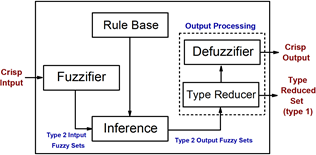Figure 1. Type-2 fuzzy logic system structure 2.1.2 Parameter update rules The determination of the unknown parameters of the antecedent and the consequent parts of the type2-fuzzy If-Then rules is crucial to design the type2–fuzzy adaptative filter. The input space in the antecedent parts is decomposed into a set of type-2 fuzzy regions, while the consequence parts are decomposed into regions which are automatically determined. The ambiguity in the Gaussian type-2 membership functions can be attributed to the center (mean) and the standard deviation. In this investigation, the Gaussian type-2 fuzzy membership functions were chosen due to their ability to uniformly estimate continuous functions, and to their ability to universal approximation . Figure 2 represents the Gaussian type-2 fuzzy membership function where the uncertainty is associated with the center (mean) and with the standard deviation (width). The Gaussian membership function can be expressed mathematically as: \tilde{\mu}(x)=\exp \left(\frac{1}{2} \frac{(x-c)^{2}}{\sigma^{2}}\right) (3) where, c: the center (mean) of the membership function; \sigma: the width (standard deviation) of the membership function; x: is the data. In this paper, uncertainty is considered both on the center (mean) c=\left[c_{1}, c_{2}\right] and on the standard deviation \sigma=\left[\sigma_{1}, \sigma_{2}\right], where c_{1}, \sigma_{1} and c_{2}, \sigma_{2} are the lower and upper bounds of the uncertainty interval, respectively. ## qqtu_pian_20201029143635.png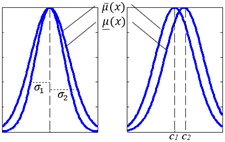Figure 2. Gaussian type-2 fuzzy membership function with: (a) uncertain standard deviation; (b) uncertain center (mean) 2.2 Teacher-learning-based optimization algorithm Rao  have proposed a new evolutionary algorithm labeled TLBO (Teaching Learning Based Optimization), which is inspired by the education process at school. This optimization algorithm is based on the impact of the teacher on the learners in the classroom. This optimization algorithm uses a population of solutions which contains a possible solution (feasible solution) of the optimization problem under consideration. Basically, this population is a group of learners. Several subjects given to the learners can be considered to be the design variables which are the parameters associated with the objective function of the optimization problem. Learner’s result is analogs to the fitness value of the feasible solution of the optimization problem. TLBO algorithm is divided into two phases: “Teacher phase” and “learner phase” which are described below: 2.2.1 Teacher phase Learning of the learners form the teacher is considered to be the first phase of the TLBO algorithm, where the teacher is considered as the highly learned and skilled person in society. For simulations, we assume that there are ‘m’ number of subjects (design variables, j=1,2, \ldots, m), ‘n’ number of learners (population size, k=1,2, \ldots, n) and M_{j}^{i} be the mean results of the students in a particular subject ‘j’ at any teaching-learning cycle (i=0,1,2 \ldots, I_{n}). A Teacher is the most experienced, knowledgeable, and highly learned person in society. To simulate this concept, the best learner (feasible solution) in the entire population is considered as a teacher. Let X_{j}^{i} be the best feasible solution of the population at the i^{t h} teaching-learning cycle and X_{T, j}^{i} denotes the j^{t h} design variable in the best feasible solution of the population at the i^{t h} teaching-learning cycle or the result of the teacher in subject j ". The difference between the result of the teacher and the mean result of the learners in subject j ' can be expressed as: D_{j}^{i}=r\left(X_{T, i}^{i}-T_{F} M_{j}^{i}\right) (4) where, X_{T, j}^{i} is the result of the best student in subject ^{\prime} j^{\prime} \cdot r is a random number in the range [0,1]. T_{F} is the teaching factor which decides the value of the mean to be changed, the value of T_{F} can be either 0 or 1. T_{F} is not a parameter of the TLBO algorithm, and is not given as an input to the algorithm and its value is randomly decided by the algorithm using the following equation: T F= round [1+ rand (0,1)\{2-1\}] (5) Based on the difference between the result of the teacher and the mean result of the learners in subject ‘j’ (D_{j}^{i}), the existing solution is updated in the teacher phase according to the following expression: X_{n e w, k, j}^{i}=X_{o l d, k, j}^{i}+D_{j}^{i} (6) X_{n e w, k}^{k} is accepted if it gives better function value than X_{\text {old}, k}^{k}. All the accepted feasible solutions are maintained and these become the input to the student phase. The student phase depends upon the teacher phase. 2.2.2 Learner phase It is the second part of the TLBO algorithm where learners increase their knowledge by interaction among themselves. A learner interacts randomly with other students for enhancing his or her knowledge. A learner (u) learns new things if the other learner (v) has more knowledge than him or her. The learning philosophy of this phase is simulated as below: Randomly select two students u and v, where their feasible solutions are X_{u}^{i} and X_{v}^{i}, respectively and then: if F\left(X_{u}^{i}\right)>F\left(X_{v}^{k}\right) X_{n e w_{S P}, u, j}^{i}=X_{u, j}^{i}+r\left(X_{u, j}^{i}-X_{v, j}^{i}\right) else X_{n e w, u}^{i}=X_{n e w, u}^{i} end if where, F(x): is a fitness function that is used to find the fitness value of a feasible solution; X_{n e w_{S P}, u, j}^{i}: is the jth design variable of the modified feasible solution in student phase at ith teaching-learning cycle and it is accepted if it gives a better function value as follows: If F\left(X_{\text {new } S P, u}^{i}\right)>F\left(X_{\text {new }, u}^{i}\right) X_{\text {new}, u}^{i}=X_{\text {new } S P, u}^{k} else X_{n e w, u}^{i}=X_{n e w, u}^{i} end if 3. Design Procedure of the TLBO-Based Type2-Fuzzy Adaptive Filter Figure 3 shows the proposed TLBO-based type-2 fuzzy adaptive filter framework: ## 3.png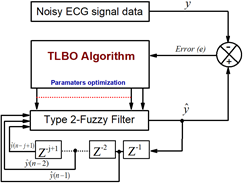Figure 3. Proposed framework of the TLBO based type-2fuzzy adaptive filter To construct the TLBO based-type-2 fuzzy adaptive filter, the following phases are used: Phase 1: Consider N type-2 fuzzy sets F_{i}^{l} for all input space intervals \left[C_{i}-, C_{i}+\right] having Gaussian type-2 fuzzy membership functions as: \mu_{F_{i}^{l}}\left(x_{i}, \mu_{P_{i}^{l}}\right)=\exp \left(-\frac{1}{2}\left(\frac{\mu_{P}^{l}-m_{i}^{l}\left(x_{i}\right)}{\sigma_{m_{i}}^{l}}\right)^{2}\right) (7) where, \mu_{F_{i}^{l}} and \mu_{P_{i}^{l}} are the upper and the lower bounds of the Gaussian membership function, respectively, with \mu_{P_{i}^{l}} \in[0,1]; \sigma_{m_{i}^{l}} is the standard deviation of the upper membership function; m_{i}^{l}\left(x_{i}\right) is the mean of the upper membership function, which is characterized by Gaussian membership function with mean M and standard deviation \sigma_{x} as follows: m_{i}^{l}\left(x_{i}\right)=\exp \left(-\frac{1}{2}\left(\frac{x_{i}-M_{i}^{l}}{\sigma_{i}^{l}}\right)^{2}\right) (8) where, l=1,2, \ldots, N, i=1,2, \ldots, n ; the filter's input is represented as x_{i}=x(k-I+1), the center of the i^{t h} membership function in the l^{t h} rule is represented as m_{i}^{l}, and the width of the i^{t h} membership function in the l^{t h} rule is represented as \sigma_{m_{i}^{l}}. Within this study the free parameters m_{i}^{l} and \sigma_{m_{i}^{l}} will be tuned using the evolutionary algorithm TLBO. The Gaussian type-2 fuzzy membership function (Eq. (7)) was chosen over triangular, trapezoidal or other shapes because it is a universal approximator that is able to uniformly estimate any continuous function in a compact set . Nevertheless, when this type of network employs the other membership function types, verification of the universal approximation capability is difficult. Additionally, in order to complete function approximation, a large number of rules are required. Phase 2: Construct a set of adjustable type2-fuzzy rules using the numerical information of the training input-output data pairs: R^{l}: If x_{i} is F_{1}^{l} and, ..., and x_{n} is F_{n}^{l} Then \hat{y}=\bar{Y}^{l} l=1,2, \ldots, N (9) \hat{y}: the desired output;where, F_{i}^{l}'s: type-2 fuzzy sets of the antecedent; \bar{Y}^{i}'s: type-2 fuzzy sets of the consequent having a singleton type-2 membership function \mu_{\bar{Y}} l; There will be a change of the membership function parameters of \mu_{F_{i}^{l}} and \mu_{\bar{Y}} l during the adaptation process. Phase 3: Through the use of the fundamental Karnik-Mendel center of sets type reduction  and the centroid defuzzification, the type-2 fuzzy filter is constructed around the set of N rules. Phase 4: Adjustment of the filter’s parameters by TLBO algorithm so that the fitness function error e between the type-2 fuzzy adaptive filter output \hat{y} and the noisy ECG signal y attains its minimum value (see Figure 3). The filter parameters that are trained are the consequence intervals \bar{Y}^{l} and the Gaussian center of the premise membership functions \mu_{F_{i}^{l}}. The objective function that is used throughout the investigation (TLBO optimization cost function) is the Mean Square Error (MSE) expressed as follows: M S E=\frac{\sum_{k=1}^{N}\left(y_{k}-\hat{y}_{k}\right)}{N}=\frac{\sum_{k=1}^{N} e_{k}^{2}}{N} (10) where, y_{k} is the actual measure, \hat{y}_{k} is its estimate, and N is the length of the data. 4. Results and Discussion proposed method to filter ECG signals. For this purpose, 200 signal segments of real ECG signals from different subjects are selected from the MIT-BIH database [50, 51]. The suggested method was simulated with N=40 rules. Therefore, the filter was structured using a set of 40 rules with 40 \times 3=120 adjustable parameters. consequently, there was two regressors to every rule and one consequence interval (2 \times 40 antecedent parameters and 1 \times 40 consequence parameters). The type-2 fuzzy adaptive filter's initial input vector x is set as x=\left[x_{1}, x_{2}\right]^{T}=[0,0]^{T} . The effectiveness of the proposed approach will be fully investigated by assessing its performance in some noisy environments. These include the typical noises associated with ambulatory ECG recordings, which involve MA and EM. These are individually selected from the MIT-BIH stress data . In addition, the proposed method will be also tested with artificially generated white Gaussian noise, which will be added to the original ECG segments with a number of input S N R levels. In general, evaluation of signal denoising methods usually involves a measure of similarity between the denoised signal and the original signal. In order to assess the obtained type-2 fuzzy filter output fit, four of the frequently used criteria from other experimental studies have been applied. Signal-to noise output ration (S N R_{\text {out}}): S N R_{\text {output}}=10 \times \log \left(\frac{\sum_{k=1}^{N}\left(x_{k}\right)^{2}}{\sum_{k=1}^{N}\left(\hat{y}_{k}-x_{k}\right)^{2}}\right) (11) Mean Square Error (M S E): M S E=\frac{1}{N} \sum_{k=1}^{N}\left(\hat{y}_{k}-x_{k}\right)^{2} (12) The SNR improvement (S N R_{i m p}): S N R_{i m p}=S N R_{\text {output}}-S N R_{\text {input}} (13) The Root Mean Square Error (RMSE): R M S E=\sqrt{\frac{1}{N} \sum_{k=1}^{N}\left(\hat{y}_{k}-x_{k}\right)^{2}} (14) where, x_{k} is the clean signal, \hat{y}_{k} is its estimate and N is the length of the data. 4.1 Denoising To evaluate the outcomes of the proposed technique, three types of noise are utilized (real and artificial noises) and added to the real ECG referenced 18177.dat, taken from the MIT-BIH database . The denoising results for different noise types are shown in Figures 4-7. The results show that with the addition of white Gaussian noise at 3dB SNR level, the denoised ECG signal is very close to the clean ECG morphology (see Figure 4). Meanwhile, Figure 5 shows that the denoised signal does not show any EMG artefacts with real MA noises at 3dB SNR level. Nevertheless, whilst the most troublesome noise is the EM, due to its ability to mimic ectopic beats, a very successful outcome is obtained with this experiment. Normally, undesired notches and EM noise are seen on the ST segment, and it is very difficult to remove them using simple bandpass filters; however, the proposed method is able to remove these motion artefacts (EM), as shown in Figure 6, whilst also preserving the signal’s diagnostic morphology information. In order to assess the proposed method’s efficiency to remove more complicated noises, all three noises (EM, MA and white Gaussian noise) are added to the same signal (18177.dat). Comparing the noised ECG with the denoised one, it can be seen that the proposed method produces a very smooth signal (see Figure 7). In what follows, the recording 18177.dat is used to evaluate the effectiveness of the proposed technique under different S N R input levels of real EM and MA, and artificial WGN noises where severe distortion occurs. Fuzzy filter output is assessed for each noise power case by using the criteria formulas (11)-(14). The SNRoutput, SNRimp, MSE and RMSE generated by the proposed framework for WGN, MA and EM versus different SNRinput(0dB to 10dB) are shown in Figure 8. These results show that the method achieves positive results in all different noise environments for a number of SNRinputlevels. This can be seen in Figure 8 where the slope of the different criteria, particularly the SNRoutput, are not flat and there is an obvious increase of the SNRoutput with a corresponding decrease in SNRinput. So, this confirms that the framework is capable of optimally filter the ECG signal even in the presence of artefacts such as WGN, MA, and EM and with severe distortions. Next, the proposed type-2 fuzzy adaptive filter is applied to a series of real ECG signals to further test the validity of the approach. The considered records are 100.dat, 103.dat, 200.dat and 208.dat taken from the MIT-BIH arrhythmia database. The noisy ECG signal is constructed by adding 5dB Gaussian white noise (taking into account the real existing noise in the records). Visual inspections of Figures 9, 10, 11 and 12 show the filtering efﬁciency. 4.2 Comparison The performances of the introduced filter will be compared to the results obtained by recently published methods in ECG signal denoising. Tables 1-4 give detailed results obtained by comparing the proposed method with the adaptive dual threshold filter and discrete wavelet transform (ADTF-DWT) method . The results given by Jenkal et al.  were validated by the works of several authors who compared the ADTF-DWT with the parallel-type fractional zero-phase filtering (FZP) , the Reimann- Liouville (RL) integrator , and the zero-phase average window filter (AZP) . The EMG artefact comparison results are shown in Table 1. The noises are simulated in the same manner as the experiment described in the study . Denoising comparison of 5dB white Gaussian noise from the selected entries of the MIT-BIH arrhythmia database  is shown in Table 2. The original paper  compares its results with a number of different methods, including the adaptive dependent wavelet thresholding technique (ADWT) and the multi-adaptive bionic-wavelet transform (MABWT) . Tables 3 and 4 show the results of the comparison between the proposed method and some benchmark methods at the same levels of WGN. This survey comparison shows that the results obtained from the proposed filter are much better than those obtained from the other benchmark methods, namely the ADTF-DWT, RL, AZP, FZP, ADWT, MABWT methods as detailed by Jenkal et al. . Indeed, the results show that the proposed method shows an increased SNR improvement and a reduced mean square error (MSE). In order to generate further validity in our results, we also compared the proposed approach performance against another recently published method  that is based on the adaptive Fourier decomposition (AFD). In , the authors made comparisons of the AFD based method with a number of other EMD and EEMD based methods described by Chang et al. . The results of the comparison between the AFD method and the proposed method are presented in Table 5. They show that again, the introduced method performs better in terms of MSE criterion (These results that are presented in Table 5 are calculated with signal magnitude that has been increased by 200 times from its original value). ## 4.png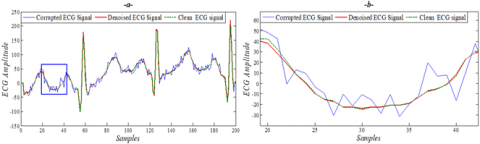Figure 4. (a) Typical filtering of the proposed method for the record 18177.dat; with an added WGN at 3dB SNR level (b) Zoomed segment of denoised ECG signal ## 5.png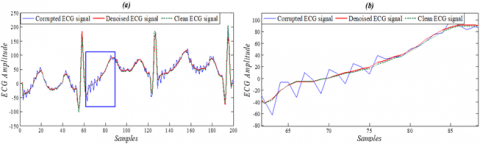Figure 5. (a) Typical filtering of the proposed for the Record 18177.dat; with muscle artifact (MA) at 3dB SNR level (b) Zoomed segment of denoised ECG signal ## 6.png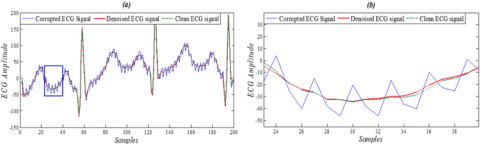Figure 6. (a) Typical filtering of the proposed method for the Record 18177.dat; with electrode motion artifact (EM) at 3dB SNR level (b) Zoomed segment of denoised ECG signal ## 7.png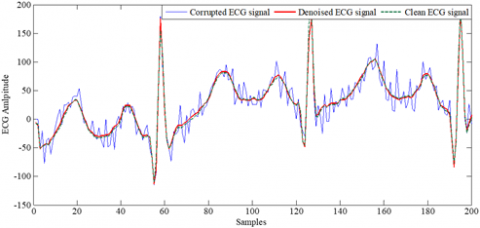Figure 7. Typical filtering of the proposed method for the record 18177.dat with the three noises (WGN+MA+EM) ## 8.png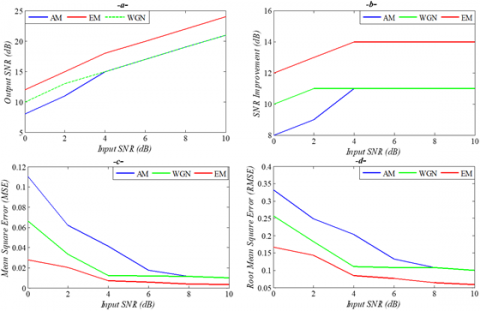Figure 8. Measures performances of the proposed method versus different input SNRs for WGN, EM and AM noise: (a) SNRoutput(b) SNR improvement (c) MSE (d) RMSE ## 9.png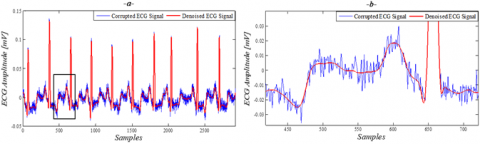Figure 9. (a) Typical filtering of the proposed method for the Record 100.dat with WGN at 5dB SNR level (b) Zoomed segment of denoised ECG signal ## 10.pngFigure 10. (a) Typical filtering of the proposed method for the Record 103.dat with WGN at 5dB SNR level (b) Zoomed segment of denoised ECG signal ## 11.pngFigure 11. (a) Typical filtering of the proposed method for the Record 200.dat with WGN at 5dB SNR level (b) Zoomed segment of denoised ECG signal ## 12.png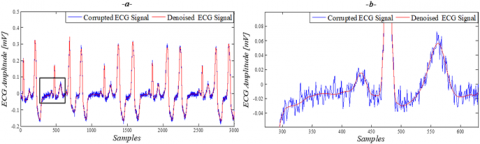Figure 12. (a) Typical filtering of the proposed method for the Record 208.dat with WGN at 5dB SNR level (b) Zoomed segment of denoised ECG signal Table 1. Comparison of the denoising results of the EMG artefact in the record 115.dat  Criteria RL  AZP  FZP  ADTF-DWT  Proposed Method SNRoutput 6.830 11.820 13.680 15.590 29.220 MSE 0.07050 0.02230 0.01460 0.01460 0.00810 Table 2. Comparison of the denoising results of the WGN noise at 5dB in some record taken from the MIT-BIH arrhythmia database  Record N° Criteria ADTF-DWT  Proposed Method 100.dat MSE 0.0044 0.00310 RMSE 0.0660 0.05560 SNRimp 9.7000 19.2200 101.dat MSE 0.0042 0.00230 RMSE 0.0400 0.04790 SNRimp 10.230 19.6100 103.dat MSE 0.0058 0.00280 RMSE 0.0760 0.05290 SNRimp 9.1000 19.6800 113.dat MSE 0.0088 0.00190 RMSE 0.0930 0.04350 SNRimp 9.3300 21.0500 115.dat MSE 0.0122 0.00730 RMSE 0.1100 0.08540 SNRimp 9.4500 15.4300 117.dat MSE 0.0283 0.00890 RMSE 0.1680 0.09430 SNRimp 9.3400 13.0100 119.dat MSE 0.0459 0.00880 RMSE 0.2140 0.09380 SNRimp 8.1300 13.9500 122.dat MSE 0.0411 0.00630 RMSE 0.2020 0.07930 SNRimp 8.0700 16.7400 Table 3. Comparison of the denoising results of the WGN (5dB) in the record 101.dat  Criteria ADWT  ADTF-DWT  Proposed Method MSE 0.0050 0.0042 0.0023 RMSE 0.0720 0.0640 0.0479 SNRimp 9.0980 10.2300 19.6100 Table 4. SNRimp comparison of denoising results of the WGN (5dB) in some record taken from MIT-BIH database  Record N° ADWT  MABWT  ADTF-DWT  Proposed Method 100.dat 9.90 7.80 9.70 19.22 101.dat 9.09 6.90 10.23 19.61 103.dat 7.13 7.70 9.10 19.68 113.dat 7.82 7.90 9.33 21.05 115.dat 7.19 7.80 9.45 15.43 117.dat 8.62 7.90 9.34 13.01 119.dat 7.27 7.60 8.13 13.95 122.dat 7.86 6.90 8.07 16.74 Table 5. Comparison between filtered results using the AFD, EEMD, EMD and the proposed method with noisy signals at 10dB SNR level in terms of MSE criterion  MIT-BIH Record N° EMD  EEMD  AFD  Proposed Method 101.dat 126.9 97.4 36.0 24.55 102.dat 83.3 60.0 32.6 19.32 103.dat 189.4 147.0 76.0 44.93 104.dat 151.6 109.5 55.7 28.22 105.dat 180.6 128.1 73.6 48.52 106.dat 245.6 192.5 98.9 57.82 107.dat 771.6 574.9 572.6 88.98 108.dat 103.2 76.9 24.0 14.45 109.dat 237.2 179.7 112.1 98.20 201.dat 67.1 38.6 38.3 26.82 202.dat 131.0 76.3 28.4 25.32 203.dat 279.7 206.5 321.3 45.70 205.dat 72.5 55.0 29.5 19.22 207.dat 129.7 99.9 93.9 53.32 208.dat 361.2 232.0 199.2 67.32 209.da 140.3 103.3 60.8 45.22 Table 6. Comparison between the filtered results of MP-EKF, EKS, EKF and the proposed method in the presence of artificial and real noises in terms of MSEPWRD index  MSEPWRD (\operatorname{mean} \mp S D)(m v) Noise type Method 0 dB -1dB -3 dB -5 dB Gaussian White Noise MP-EKF 1.2840±0.2250 1.3290±0.2240 1.4340±0.2310 1.5520±0.2420 EKS 1.3580±0.1800 1.4580±0.1960 1.6780±0.2370 1.9239±0.2880 EKF 1.6770±0.1830 1.8240±0.2000 2.1580±0.2420 2.5520±0.2970 Proposed Method 0.3860±0.0470 0.4584±0.0780 0.5176±0.0597 0.5547±0.3210 Real Muscle Artifact MP-EKF 1.4680±0.1990 1.5520±0.2000 1.7470±0.2230 1.9870±0.2550 EKS 2.9330±0.4550 3.2470±0.5140 3.9920±0.6560 4.9180±0.8360 EKF 3.0570±0.4730 3.3930±05340 4.1880±0.6810 5.1790±0.8660 Proposed Method 0.3932±0.0599 0.4530±0.0499 0.5877±0.0546 0.6932±0.0385 ## 13.png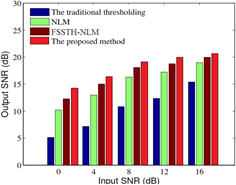Figure 13. Comparative results in the terms of SNRoutput ## 14.pngFigure 14. Comparative results in the terms of RMSE In order to further assess the efficiency of the proposed technique, Figures 13 and 14 show the comparisons based on SNRoutput and RMSE, for the proposed method and the new approaches labeled (FSSTH-NLM and NLM)  applied on a synthetic ECG signal simulated using Open-source electrophysiological Toolbox (OSET) , distorted by many noises of various SNRinput levels. By a visual inspection of Figures 13 and 14 we can see that the proposed approach outperforms the other three techniques by achieving higher SNRoutputand lower RMSE. Following this analysis, the proposed method will be further tested against a model-based method described by Hesar and Mohebbi , which presents an ECG signal denoising method labelled MPEKF. This method uses an automatic particle weighting strategy and an extended Kalman filter. The proposed method is compared to our method in terms of an ECG diagnostic distortion measure called the Multi-Scale Entropy based Weighted Distortion Measure [15, 53]. We use a similar method as that utilized by Hesar and Mohebbi  to calculate this measure. A weighted percentage root square difference (WPRD) is used as a metric, which is generated by comparing the original sub-band wavelet coefficient with the filtered signals. It uses weights that are the same as the corresponding sub-band’s multi-scale entropies. Using this measure allows to achieve an accurate representation of the distortion of the filtered signal at all sub-bands [15, 53]. It was necessary to decompose both signals using wavelet filters up to L levels in order to calculate this metric. Both the sampling frequency and the nature of the signal dictate the number of levels. An accurate ECG trace will include a sharp QRS complex segment and the slow P and T waves, therefore, effective decomposition of an ECG should display an effective representation of the detail coefficients of the QRS complexes and the approximation coefficients of the P and T waves. As such, Daubechies 9/7 bi-orthogonal wavelet filter  was used for decomposition purposes. This led us to choose L=4 for sampling frequency of 128Hz . The multiscale entropy-based weighted PRD measure is defined as: M S E W P R D=w_{A_{L}} \times$$\left(\sqrt{\frac{\sum_{k=1}^{N_{A_L}}\left[A_{L}(k)-\tilde{A}_{L}\right]^{2}}{\sum_{k=1}^{N_{A_L}}\left[A_{L}(k)\right]^{2}}} \times 100\right)$$+\sum_{j=1}^{L} w_{D_{j}} \times\left(\sqrt{\frac{\sum_{k=1}^{N_{D} j}\left[D_{j}(k)-\tilde{D}_{j}(k)\right]^{2}}{\sum_{k=1}^{N_{D} j}\left[D_{j}(k)\right]^{2}}} \times 100\right)$     (15)

where: $w_{A_{L}}$ denotes the weight of the Lth approximation band; $W_{D j}$ denotes the weight of the jth level details sub band; AL and $\tilde{A}_{L}$ denote the Lth approximation band coefficients of the original and the denoised signals, respectively; and $D_{j}$ and $\widetilde{D}_{i}$ denote the jth details band coefficients of the original and the denoised signals, respectively. The weights are: $w_{A_{L}}$ and $w_{D j}$.

The results of the comparison between our method and that of MP-EKF, EKF and EKS, as detailed in Hesar and Mohebbi study , using a MSEWPRD with various input SNR levels, are shown in Table 6. These results were generated by determining the MSEWPRDs of 200 filtered ECG segments that were selected from the MIT-BIH database. However, the chosen segments used in our algorithm can be not the same as those segments used by Hesar and Mohebbi .

4.3 Discussion

Using a set of type2-fuzzy If-Then rules, we constructed a type-2 fuzzy adaptive filter that was able to adaptively change in order to reduce the criterion functions to their minimum values. The obtained results show that the proposed filter is highly effective in denoising the ECG signal; however, the efficiency of the method’s performance is determined by the number of fuzzy adaptive filter rules and the parameters of the optimization algorithm. The effectiveness of the investigated technique was compared to simulation results from both model and non-model-based methods using SNR, MSE and RMSE criteria, with the SNR being the power ratio between signal and noise. As such, a larger SNR indicates reduced background noise and, therefore, an increase signal denoising performance. Conversely, the MSE is a tracking accuracy measurement of the filtered signal compared to the original signal, therefore, the performance of the signal information retention is better when the MSE is smaller and the RMSE is used to calculate the variance between the real signal and its estimate. A smaller RMSE leads to a smaller difference. Figures 4-14 show that the proposed method is very effective when we are dealing with real noises, white Gaussian noise and their combination with different SNRinput levels. As such, it can be deduced that this method is both accurate and robust. Furthermore, by filtering the EMG artefact and comparing the outcomes with those of other benchmark methods, it can be seen that the proposed filter is statistically more effective than FSSTH-NL, ADTF-DWT and other methods described by Bing et al. [6-11]. Comparison results between the proposed method’s denoising performance using white Gaussian noise of 5dB SNR level with the ADTF-DWT and other methods [7-12] are shown in Tables 2-4. These results show that the proposed method has improved results in terms of MSE, RMSE and SNRimp when compared to ADTF-DWT, ADWT and MABWT methods. Furthermore, the proposed approach provides an important solution for the problem of high-density noise as shown with the results of the 5dB white Gaussian noise. Table 5 displays a deeper comparison of the filtered results under MSE performance using the proposed approach and the AFD, EEMD and EMD methods detail [9-13]. These results also show that the proposed approach is again, more effective. Moreover, the results displayed in Table 6 shows the comparison between the proposed method and MP-EKF and EKF/EKS  using the MSEWPRD on two types of noise with four different input SNRs (0dB, -1dB, -3dB and -5dB). Notice that the MP-EKF and EKF/EKS have higher MSEWPRDs for each noise type at all SNRinputlevels compared to the proposed method indicating that our method is more effective than the MP-EKF and EKF/EKS in preserving the diagnostic information and morphology of the ECG signals.

5. Conclusion

In this paper, a novel adaptive type-2 fuzzy filter for ECG signal denoising was presented. The main blocks used in the filter were a type2-fuzzy system and a TLBO optimization. TLBO algorithm was designed to updating a type-2 fuzzy system parameters. The proposed filter was utilized to denoise ECG signals and was found to be highly effective at removing electrode motion noise, EMG noise and white Gaussian noise. In addition, a number of comparisons were made between the performances of the proposed approach with a number of other methods that have been presented in previously published studies. The results of these comparisons show that our filtering technique has better outcomes with a higher SNRoutput and an improved SNRimp, MSE, RMSE and MSEPWRD than the other model based and non-model-based methods detailed in the researches [6, 7, 9, 13, 15]. additionally, the proposed filter was able to preserve the morphology of the ECG signal and maintain the diagnostic performance.

The type2 fuzzy adaptive filter proposed in this paper may open up new horizons for efficient denoising of ECG signals. Therefore, as future work, we suggest further development of the proposed filter to (1) Minimize the processing time and (2) Increase the robustness:

(1) For the first point, it is known that the main problem with the type-2 fuzzy systems is the type reduction process which is computationally complicated, especially when there are many MFs and the rule base is large, therefore as a perspective of this work we propose to reduce the computational burden, using a faster type-reduction method. Several algorithms are being developed for this purpose, including the modified enhanced Karnik-Mendel (MEKM) method, enhanced Karnik-Mendel (EKM) method, the enhanced iterative algorithm with stop condition (EIASC) method and many other methods reported by Tai et al. .

(2) For the second point, we propose to use other optimization algorithms such as BBO, IWO, CMA. ES, SCE. UA, SFLA, PSO and even combine them in one algorithm in order to consider the advantage of every one and create a more robust optimization.

References

 Fischbach, M. (2002). Guide pratique du cardiaque: pré-vention et suivi. Frison-Roche.

 Venes, D. (2017). Taber's cyclopedic medical dictionary. FA Davis.

 Betts, J.G., Desaix, P., Johnson, J.E., Korol, O., Kruse, D., Poe, B. (2013). Anatomy & Physiology: OpenStax College. Rice University, USA.

 Jenkal, W., Latif, R., Toumanari, A., Dliou, A., El B’charri, O., Maoulainine, F.M.R. (2016). QRS detection based on an advanced multilevel algorithm. International Journal of Advanced Computer Science and Applications, 1(7): 253-260. https://doi.org/10.14569/IJACSA.2016.070135

 Khaing, A.S., Naing, Z.M. (2011). Quantitative investigation of digital filters in electrocardiogram with simulated noises. International Journal of Information and Electronics Engineering, 1(3): 210-216. https://doi.org/10.7763/IJIEE.2011.V1.33

 Bing, P., Liu, W., Wang, Z., Zhang, Z. (2020). Noise reduction in ECG signal using an effective hybrid scheme. IEEE Access, 8: 160790-160801. https://doi.org/10.1109/ACCESS.2020.3021068

 Jenkal, W., Latif, R., Toumanari, A., Dliou, A., El B’charri, O., Maoulainine, F.M. (2016). An efficient algorithm of ECG signal denoising using the adaptive dual threshold filter and the discrete wavelet transform. Biocybernetics and Biomedical Engineering, 36(3): 499-508. https://doi.org/10.1016/j.bbe.2016.04.001

 Nguyen, P., Kim, J.M. (2016). Adaptive ECG denoising using genetic algorithm-based thresholding and ensemble empirical mode decomposition. Information Sciences, 373: 499-511. https://doi.org/10.1016/j.ins.2016.09.033

 Wang, Z., Wan, F., Wong, C.M., Zhang, L. (2016). Adaptive Fourier decomposition-based ECG denoising. Computers in Biology and Medicine, 77: 195-205. https://doi.org/10.1016/j.compbiomed.2016.08.013

 Wang, J., Ye, Y., Pan, X., Gao, X. (2015). Parallel-type fractional zero-phase filtering for ECG signal denoising. Biomedical Signal Processing and Control, 18: 36-41. https://doi.org/10.1016/j.bspc.2014.10.012

 Wang, J., Ye, Y., Pan, X., Gao, X., Zhuang, C. (2014). Fractional zero-phase filtering based on the Riemann-Liouville integral. Signal Processing, 98: 150-157. https://doi.org/10.1016/j.sigpro.2013.11.024

 Awal, M.A., Mostafa, S.S., Ahmad, M., Rashid, M.A. (2014). An adaptive level dependent wavelet thresholding for ECG denoising. Biocybernetics and Biomedical Engineering, 34(4): 238-249. https://doi.org/10.1016/j.bbe.2014.03.002

 Chang, K.M., Liu, S.H. (2011). Gaussian noise filtering from ECG by wiener filter and ensemble empirical mode decomposition. Journal of Signal Processing Systems, 64(2): 249-264. https://doi.org/10.1007/s11265-009-0447-z

 McSharry, P.E., Clifford, G.D., Tarassenko, L., Smith, L.A. (2003). A dynamical model for generating synthetic electrocardiogram signals. IEEE Transactions on Biomedical Engineering, 50(3): 289-294. https://doi.org/10.1109/tbme.2003.808805

 Hesar, H.D., Mohebbi, M. (2016). ECG denoising using marginalized particle extended Kalman filter with an automatic particle weighting strategy. IEEE Journal of Biomedical and Health Informatics, 21(3): 635-644. https://doi.org/10.1109/JBHI.2016.2582340

 Sameni, R., Shamsollahi, M.B., Jutten, C., Clifford, G.D. (2007). A nonlinear Bayesian filtering framework for ECG denoising. IEEE Transactions on Biomedical Engineering, 54(12): 2172-2185. https://doi.org/10.1109/TBME.2007.897817

 Sayadi, O., Shamsollahi, M.B. (2008). ECG denoising and compression using a modified extended Kalman filter structure. IEEE Transactions on Biomedical Engineering, 55(9): 2240-2248. https://doi.org/10.1109/10.1109/TBME.2008.921150

 Chafaa, K., Ghanai, M., Benmahammed, K. (2007). Fuzzy modelling using Kalman filter. IET Control Theory & Applications, 1(1): 58-64. https://doi.org/10.1049/iet-cta:20050268

 Sumathi, S., Paneerselvam, S. (2010). Computational Intelligence Paradigms: Theory & Applications Using MATLAB. CRC Press.

 dos Santos Coelho, L., Herrera, B.M. (2006). Fuzzy modeling using chaotic particle swarm approaches applied to a yo-yo motion system. 2006 IEEE International Conference on Fuzzy Systems, Vancouver, BC, pp. 2293-2298. https://doi.org/10.1109/FUZZY.2006.1682018

 Li, Y., Tang, Y.G. (2010). Chaotic system identification based on a fuzzy Wiener model with particle swarm optimization. Chinese Physics Letters, 27(9): 090503.

 Abiyev, R.H., Kaynak, O., Alshanableh, T., Mamedov, F. (2011). A type-2 neuro-fuzzy system based on clustering and gradient techniques applied to system identification and channel equalization. Applied Soft Computing, 11(1): 1396-1406. https://doi.org/10.1016/j.asoc.2010.04.011

 Zhang, H., Luo, Y., Liu, D. (2006). A new fuzzy identification method based on adaptive critic designs. International Symposium on Neural Networks, 804-809. https://doi.org/10.1007/11759966_118

 Olivas, F., Valdez, F., Castillo, O., Melin, P. (2016). Dynamic parameter adaptation in particle swarm optimization using interval type-2 fuzzy logic. Soft Computing, 20(3): 1057-1070. https://doi.org/10.1007/s00500-014-1567-3

 Sanchez, M.A., Castillo, O., Castro, J.R. (2015). Information granule formation via the concept of uncertainty-based information with interval type-2 fuzzy sets representation and Takagi–Sugeno–Kang consequents optimized with Cuckoo search. Applied Soft Computing, 27: 602-609. https://doi.org/10.1016/j.asoc.2014.05.036

 Valdez, F., Vazquez, J.C., Melin, P., Castillo, O. (2017). Comparative study of the use of fuzzy logic in improving particle swarm optimization variants for mathematical functions using co-evolution. Applied Soft Computing, 52: 1070-1083. https://doi.org/10.1016/j.asoc.2016.09.024

 Perez, J., Valdez, F., Castillo, O., Melin, P., Gonzalez, C., Martinez, G. (2017). Interval type-2 fuzzy logic for dynamic parameter adaptation in the bat algorithm. Soft Computing, 21(3): 667-685. https://doi.org/10.1007/s00500-016-2469-3

 The MIT-BIH noise stress test database available at: https://physionet.org/physiobank/database/nstdb/, accessed on Dec. 25, 2019.

 Zadeh, L.A. (1975). The concept of a linguistic variable and its application to approximate reasoning-I. Information Sciences, 8(3): 199-249. https://doi.org/10.1016/0020-0255(75)90036-5

 Xie, W.F., Rad, A.B. (1999). Fuzzy on-line identification of SISO nonlinear systems. Fuzzy Sets and Systems, 107(3): 323-334. https://doi.org/10.1016/S0165-0114(97)00297-2

 Johansen, T.A., Babuska, R. (2003). Multiobjective identification of Takagi-Sugeno fuzzy models. IEEE Transactions on Fuzzy Systems, 11(6): 847-860. https://doi.org/10.1109/TFUZZ.2003.819824

 Lee, S.B., Yen, G.G. (2004). Analysis of Takagi-Sugeno fuzzy models in system identification for model-based control. Control and Intelligent Systems, 32(2): 69-79.

 Li, Z., Zhou, Y., Bao, R. (2019). An image classification method based on optimized fuzzy bag-of-words model. Traitement du Signal, 36(3): 239-244. https://doi.org/10.18280/ts.360306

 Wang, L.X., Mendel, J.M. (1993). Fuzzy adaptive filters, with application to nonlinear channel equalization. IEEE Transactions on Fuzzy Systems, 1(3): 161-170. https://doi.org/10.1109/91.236549

 Wang, L.X., Mendel, J.M. (1992). Fuzzy basis functions, universal approximation, and orthogonal least-squares learning. IEEE Transactions on Neural Networks, 3(5): 807-814. https://doi.org/10.1109/72.159070

 Kosko, B. (1994). Fuzzy systems as universal approximators. IEEE Transactions on Computers, 43(11): 1329-1333. https://doi.org/10.1109/12.324566

 Karnik, N.N., Mendel, J.M., Liang, Q. (1999). Type-2 fuzzy logic systems. IEEE Transactions on Fuzzy Systems, 7(6): 643-658. https://doi.org/10.1109/91.811231

 Mendel, J.M. (2000). Uncertainty, fuzzy logic, and signal processing. Signal Processing, 80(6): 913-933. https://doi.org/10.1016/S0165-1684(00)00011-6

 Khanesar, M.A., Kayacan, E., Teshnehlab, M., Kaynak, O. (2011). Analysis of the noise reduction property of type-2 fuzzy logic systems using a novel type-2 membership function. IEEE Transactions on Systems, Man, and Cybernetics, Part B (Cybernetics), 41(5): 1395-1406. https://doi.org/10.1109/TSMCB.2011.2148173

 Biglarbegian, M., Melek, W.W., Mendel, J.M. (2010). Design of novel interval type-2 fuzzy controllers for modular and reconfigurable robots: Theory and experiments. IEEE Transactions on Industrial Electronics, 58(4): 1371-1384. https://doi.org/10.1109/TIE.2010.2049718

 Chafaa, K., Saidi, L., Ghanai, M., Benmahammed, K. (2006). Direct adaptive type-2 fuzzy control for nonlinear systems. International Journal of Computational Intelligence and Applications, 6(3): 389-411. https://doi.org/10.1142/S1469026806001897

 Tan, D.W.W.W. (2006). A simplified type-2 fuzzy logic controller for real-time control. ISA Transactions, 45(4): 503-516. https://doi.org/10.1016/S0019-0578(07)60228-6

 Maldonado, Y., Castillo, O., Melin, P. (2014). Embedded average of an interval type-2 fuzzy systems for applications in FPGAs. Intelligent Automation & Soft Computing, 20(2): 183-199. https://doi.org/10.1080/10798587.2013.863531

 Karnik, N.N., Mendel, J.M. (1998). Introduction to type-2 fuzzy logic systems. 1998 IEEE International Conference on Fuzzy Systems Proceedings. IEEE World Congress on Computational Intelligence (Cat. No.98CH36228), Anchorage, AK, USA, pp. 915-920. https://doi.org/10.1109/FUZZY.1998.686240

 Salaken, S.M., Khosravi, A., Nahavandi, S. (2016). Modification on enhanced Karnik–Mendel algorithm. Expert Systems with Applications, 65: 283-291. https://doi.org/10.1016/j.eswa.2016.08.055

 Tai, K., El-Sayed, A.R., Biglarbegian, M., Gonzalez, C.I., Castillo, O., Mahmud, S. (2016). Review of recent type-2 fuzzy controller applications. Algorithms, 9(2): 39. https://doi.org/10.3390/a9020039

 Chafaa, K., Saidi, L., Ghanai, M., Benmahammed, K. (2007). Indirect adaptive interval type-2 fuzzy control for nonlinear systems. International Journal of Modelling, Identification and Control, 2(2): 106-119. https://doi.org/10.1504/IJMIC.2007.014623

 Wang, L.X. (1992). Fuzzy systems are universal approximators. [1992 Proceedings] IEEE International Conference on Fuzzy Systems, San Diego, CA, USA, pp. 1163-1170. https://doi.org/10.1109/FUZZY.1992.258721

 Rao, R.V. (2016). Teaching-learning-based optimization algorithm. Teaching Learning Based Optimization Algorithm, 9-39. https://doi.org/10.1007/978-3-319-22732-0_2

 The MIT-BIH arrhythmia database available at: https://www.physionet.org/physiobank/database/mitdb/, accessed on Dec. 25, 2019.

 The MIT-BIH normal sinus rhythm database available at: https://physionet.org/physiobank/database/nsrdb/, accessed on Dec. 25, 2019.

 Tian, X., Li, Y., Zhou, H., Li, X., Chen, L., Zhang, X. (2016). Electrocardiogram signal denoising using extreme-point symmetric mode decomposition and nonlocal means. Sensors, 16(10): 1584. https://doi.org/10.3390/s16101584

 Manikandan, M.S., Dandapat, S. (2008). Multiscale entropy-based weighted distortion measure for ECG coding. IEEE Signal Processing Letters, 15: 829-832. https://doi.org/10.1109/LSP.2008.2007620

 Antonini, M., Barlaud, M., Mathieu, P., Daubechies, I. (1992). Image coding using wavelet transform. IEEE Transactions on Image Processing, 1(2): 205-220. https://doi.org/10.1109/83.136597

 Manikandan, M.S., Dandapat, S. (2007). Wavelet energy based diagnostic distortion measure for ECG. Biomedical Signal Processing and Control, 2(2): 80-96. https://doi.org/10.1016/j.bspc.2007.05.001